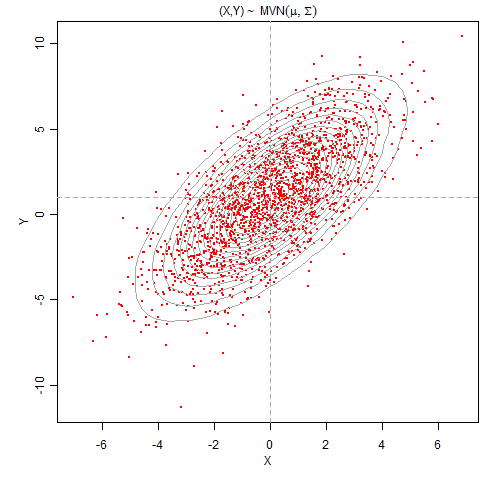Sweave本身做不到缓存（或者很难做到），但由于R语言的扩展性以及一些疯子和天才的存在，我们可以通过R的一些附加包来完成缓存。这里的缓存有两种（不关心细节的读者可以略过）：

## 如果存在，就加载
if (file.exists('x.RData')) {
}
## 如果当前工作环境中不存在x，那么就重新创建它
if (!exists('x')) {
x = rnorm(100000)
}


R对象的缓存可以通过cacheSweave包（作者Roger D. Peng）实现，它真正采用的方法比上面的伪代码当然要高级（比如用代码的MD5值作数据库名等），不过用户可以不必关心这其中的细节。这种“当需要时才加载”的方式在术语上叫“延迟加载”，即Lazy Load，这在R里面也很常见，比如很多R包的数据都是采用延迟加载的方式（加载包的时候并不立刻加载数据，而是用data(name)在需要的时候加载）。

\beginpgfgraphicnamed{graph-output-1}
\input{graph-output-1.tikz}
\endpgfgraphicnamed


pgfSweave从R的层面上可以输出这样的代码段，并生成一个shell脚本来编译图形为PDF；而pgfSweave也会尝试根据R代码的MD5来判断一段画图的代码是否需要重复运行——如果R代码没有变化，那么图形就不必重制。这是自然而然的“缓存”。对了，pgfSweave对Sweave的图形输出作了扩展，新增了tikz输出，这是通过另一个R包tikzDevice实现的（同样两个作者），这个包可以将R图形录制为tikz绘图代码。pgfSweave依赖于cacheSweave包，把它缓存R对象的机制也引入进来，所以我们可以不必管cacheSweave，把精力放在pgfSweave上就可以了。

Gibbs抽样的最终目的是从一个多维分布$$f(X_1,X_2,\ldots,X_n)$$中生成随机数，而已知的是所有的条件分布$$f(X_1|X_2,\ldots,X_n)$$$$f(X_2|X_1,\ldots,X_n)$$、……、$$f(X_n|X_1,\ldots,X_{n-1})$$，并且假设我们可以很方便从这些条件分布中抽样。剩下的事情就很简单了：任意给定初始值$$x_1^{(0)},x_2^{(0)},\ldots,x_n^{(0)}$$，括号中的数字表示迭代次数，接下来依次迭代，随机生成$$x_1^{(k)} \sim f(X_1|x_2^{(k-1)},\ldots,X_n^{(k-1)})$$$$x_2^{(k)} \sim f(X_2|x_1^{(k)},\ldots,x_n^{(k-1)})$$、……、$$x_n^{(k)} \sim f(X_n|x_1^{(k)},\ldots,x_{n-1}^{(k)})$$（迭代次数$$k=1,\cdots,N$$），也就是，根据上一次的迭代结果并已知条件分布的情况下，从条件分布中生成下一次的随机数（这个算法很简单，你什么时候看明白上标下标了就明白了）。这是个串行的过程，所以无法避免循环。

$$\left[\begin{array}{c}X\\ Y\end{array}\right]\sim\mathcal{N}\left(\left[\begin{array}{c}0\\ 1\end{array}\right],\left[\begin{array}{cc} 4 & 4.2\\ 4.2 & 9\end{array}\right]\right)$$• cache=TRUE 使得文档中的R对象可以被缓存；
• external=TRUE 使得图形可以被缓存；

1. pgfSweave对图形的缓存经常有点过度，即使你改动了代码，缓存仍然不会被替换，这种情况下你可以把生成的tikz和pdf图形删掉；

2. 中文问题：tikzDevice包好像还没有完全解决中文问题，作者正在尝试我提供的中文图形，也许在未来不久的新版本中会有完全的中文支持，但目前实际上我已经在我的自动配置脚本中解决了这个问题。我们只需要保证我们的中文LyX文档用UTF-8编码就可以了——在文档选项中使用CTeX类（以及UTF8选项），并设置语言为中文、编码为Unicode (XeLaTeX) (utf8)。这种设置至少我在Windows和MikTeX下可以成功编译中文Sweave文档。目前高亮代码功能在中文文档中还不能用，但我已经向相关作者发了补丁，等CRAN管理员回来发布新版parser包之后这个问题也会解决。所以，中文用户也不必担心，一切都（将）可以使用。

3. 依旧是样式问题：细心的读者也许能发现上面的PDF文档中的图形和这里网页中的图形略有区别，PDF文档中图形的字体和正文字体是一致的，这也是pgf的优势，前文第5节曾提到过。

4. 如果使用缓存，那么代码段中的对象都无法打印出来，即使用print(dat)也无法打印dat，这是因为缓存的代码段只管是否加载对象，而无法执行进一步的操作（包括打印），对于这种情况，我们应该设计好我们的Sweave文档结构，让某些代码段作计算（并缓存），某些代码段作输出（不要缓存）。